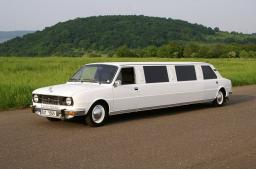Car range

Kia has an average diesel consumption of 3.9 liters per 100 kilometers. What is the range of the car for 179 euros if fuel costs 1.3 EUR/liter, if the tank has a volume of 55 liters?

d =  3530.572 km

Step-by-step explanation:Did you find an error or inaccuracy? Feel free to write us. Thank you!

Showing 1 comment:Dr MathTips to related online calculators
Need help to calculate sum, simplify or multiply fractions? Try our fraction calculator.
Check out our ratio calculator.
Do you want to convert length units?
Do you know the volume and unit volume, and want to convert volume units?